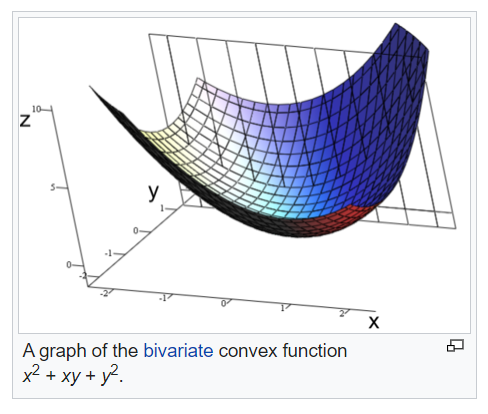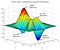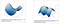# Day 53(DL) — Convex Vs Non-Convex & Saddle points in NN

The objective of any deep learning requirement is to reach the global minima where the loss will be extremely low. Till now we’ve discussed the cost function is convex in nature where we have only global minima. But for deep neural networks that is not the case, the loss function is Non-Convex. It implies, we have many local optimas and only one global minimum. Let’s compare both functions to gain better intuition.

Convex function:Fig 1 — shows convex function from wiki

Non-Convex function:Fig 2 — shows non-convex from wiki

Saddle points: In deep NNs, the cost function always tends to have saddle points where the gradients are zero(minimum value). Here the problem is plateaus, often referred to as flat surfaces where there is no change in the gradients(zero learning). If the model gets stuck in a plateau, it will slow down the learning process and sometimes there will not be any learning at all.Fig 3 — displays saddle points in the loss function from wiki

For this discussion, we will check out the working principle behind Gradient descent with momentum.

Gradient Descent with momentum: This technique greatly helps the model to reach the global minima by taking significant steps and crossing over the local minima with the momentum. It uses the exponentially weighted average to update the weights.

curr_val(exponentially weighted avg) = beta * (prev_grad) + (1-beta) * curr_grad

Bias Correction: During the initial mini-batches, there will not be much of the past data and this will create a bias since 0.9(beta) weightage is given to the history and only 0.1%(1-beta) weight assigned to current data. In order to make the learning unbiased, the entire term is divided by bias factor(1-beta^t) and this value produces effective results during the initial stage and will not have any impact in the later phase. Since ‘t’ corresponds to the iteration, initially the denominator becomes high as the iteration increases the denominator will become small(implying previous weights have more influence).

The momentum (beta) must be higher to smooth out the update because we give more weight to the past gradients. It is recommended to use the default value for β = 0.9 but if required, it can be tuned between 0.8 to 0.999.

In momentum, instead of using dW and db independently for each epoch, we take the exponentially weighted averages of dW and db.

VdW = β * VdW + (1 — β) * dW

Vdb = β * Vdb + (1 — β) * db

After calculating exponentially weighted averages, we will update our parameters.

W = W — learning rate *VdW

b = b — learning rate * Vdb

Disadvantages:

(1) It will not be able to handle saddle points where the gradient is almost the same and is not changing much.

(2) overshoots the target and has to take a lot of U-turns to reach the objective.

Recommended Reading:

https://www.youtube.com/watch?v=fODpu1-lNTw&list=PLkDaE6sCZn6Hn0vK8co82zjQtt3T2Nkqc&index=33

https://becominghuman.ai/neural-network-learning-internals-error-function-surface-non-convexity-sgd-optimization-c0209d8fdf88

AI Enthusiast